Next: MAXIMUM SATISFYING LINEAR SUBSYSTEM Up: Mathematical Programming Previous: MINIMUM PLANAR RECORD PACKING   Index

### MINIMUM RELEVANT VARIABLES IN LINEAR SYSTEM

• INSTANCE: Integer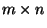-matrix, integer m-vector.

• SOLUTION: A rational n-vectorsuch that Ax=b.

• MEASURE: The number of non-zero elements in x.

• Bad News: Not in APX .

• Comment: Not approximable within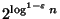for anyunless NP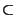QP . The above nonapproximability results are still true for the variation in which the solutions are restricted by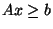instead of Ax=b. Variation in which the solution vector is restricted to contain binary numbers is NPO PB-complete and is not approximable within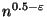for any. The complementary maximization problem, where the number of zero elements in the solution is to be maximized, and the solution vector is restricted to contain binary numbers, is NPO PB-complete and is not approximable withinfor any.

• Garey and Johnson: MP5

Viggo Kann
2000-03-20